# Physics - Electromagnetism - Force between parallel conductors and the magnetic field of a current loop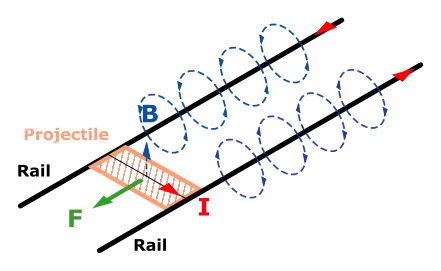Image source:## Introduction

Hello it's a me again Drifter Programming! Today we continue with Electromagnetism to get into the magnetic force between parallel current-carrying conductors and also the magnetic field of current loops/circuits. So, without further do, let's get straight into it!

## Force between parallel conductors

Last time we saw that the magnetic field around a straight-line current-carrying conductor of infinite length at a distane r is given by the equation: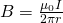Let's consider two of those straight conductors/wires that are parallel to each other with an distance r and with the same current I, which means that they generate the same magnetic field around them (perpendicular circles that can be found using the right-hand rule). As we already know current is affected by magnetic fields and the exerted force of a magnetic field to a current-carrying wire of length L is given by the equation: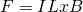Because each conductors/wire is perpendicular to the magnetic field generated by the other conductor/wire the angle between them is θ = 90degrees which means that sinθ = 1 and so (when getting into magnitude form):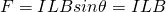By substituting B into the expression of the Force we get: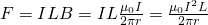When the wires have different currents (I1 and I2) then we of course would get:where the forces applied to each conductor/wire are of course equal in magnitude, but opposite in direction (each current "occurs" either in the magnetic field or in the actual force equation), which of course is true because of Newton's third law! The direction of this force of course depends on the direction of the currents (sign), which means that the force is attractive on currents in the same direction and repulsive on currents that flow in opposite directions.

Something worth noting is the force per unit of length (F/L) which is: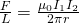Note that the official operational definition of the ampere unit (A) is based on this force between current-carrying wires. Two parallel wires separated by 1 meter and carrying 1 ampere of current have a force per meter which is: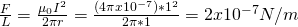Here the official definition of the ampere:

One ampere of current through each of two parallel conductors of infinite length, separated by one meter in empty space free of other magnetic fields, causes a force of exactly 2×10−7N/m  on each conductor.

## Magnetic field of a current loop

Last time we talked about the Biot-Savart law. Today we will finally use it to "prove" the equations for the magnetic field of current loops. Knowing how we get to two of the main equations used at such loops you will understand how we apply this law in practice. So, let's get started!

Let's consider a current-carrying loop of wire. The magnitude and direction of the magnetic field produced are both very complex. Biot-Savart's law tells us that magnetic field of a current element is: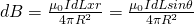Let's suppose that the loop of wire is symmetrical (circle) and so has a radius R. All the points have an angle of 90 degrees which means that the distance becomes a constant. This means that the only thing that gets integrated is dL which of course gets integrated around the total circumference (2πR) of the circle. By considering that we want to calculate the magnetic field at the center of the current loop we have: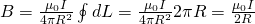You can clearly see that this equation is very similar to the equation for the straight-wire! But also note that this equation is only valid for the center of the current loop!

To create larger/stronger magnetic fields we mostly add-up more of those loops of wires. The magnetic field of N-loops (because of the superposition principle) of course will be-times what is true for one of them if we consider them all being equivalent. This means that the magnetic field at the center of N-loops of wire is given by: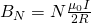Also note that the magnetic field's strengh and distance are inverse proportional. This means that the magnetic field is stronger for smaller fields, because the current at larger loops is farther away from the center, where we calculate the actual magnitude.

The magnetic field of a solenoid which is a long coil of wire (many loops) and is not "flat" like the previous cases is proven to have a magnetic field inside given by:where:

• μo is the permeability constant
• n is the number of loops per unit of length (N/L)
• I is the current

Let's now consider the same symmetrical wire as before, but this time we don't want to calculate the magnetic field at the center, but along the axis...From Biot-Savart's law and Euclid's law (r^2 = y^2 + R^2) where we are considering the distance from the center being y we have: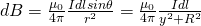The angle of course again is θ = 90 which makes the "sinθ" component '1'.

At the axis we have many magnetic fields affecting a "test" charge. More specifically, there is an magnetic field dB' due to the current element Idl ' which has an opposite direction to the "previous" current Idl. This means that the components dB and dB' which are perpendicular to the y-axis cancel each other out! So, when calculating the magnetic field we only have to consider the components along the y-axis, cause the components perpendicular sum to zero in pairs! From all that we now end up with: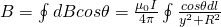cause y, R and θ are constant for all dl elements.

The element cosθ can be replaced by using the following trigonometric equation: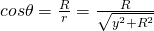This, together with the previously used integral:Now gives us the final equation which is:By setting y =0 we should find the previously calculated expression for the center! Let's check this: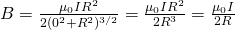:)

## Summary

To summarize really quick, the force between two parallel currents I1 and I2, separated by distance r is has a magnitude per unit of length given by the equation:and this force is attracitve on currents that flow in the same direction and repulsive for currents that flow in opposite directions!

The magnetic field of current loops is more complex and depends a lot on the observation point or point of interest. Today we saw two main cases:

• Field at Center of current loop
• Field on Axis of current loop

The equations for each "case" are:## REFERENCES:

Mathematical equations that I had in this post where drawn using quicklatex!

### Electric fields:

Getting into Electromagnetism -> electromagnetim, electric charge, conductors, insulators, quantization

Coulomb's law with examples -> Coulomb's law, superposition principle, Coulomb constant, how to solve problems, examples

Electric fields and field lines -> Electric fields, Solving problems around Electric fields and field lines

Electric dipoles -> Electric dipole, torque, potential and field

Electric charge and field Exercises -> examples in electric charges and fields

### Electric flux:

Electric flux and Gauss's law -> Electric flux, Gauss's law

Applications of Gauss's law (part 1) -> applying Gauss's law, Gauss applications

Applications of Gauss's law (part 2) -> more Gauss applications

Electric flux exercises -> examples in electric flux and Gauss's law

### Electric potential:

Electric potential energy -> explanation of work-energy, electric potential energy

Calculating electric potentials -> more stuff about potential energy, potential, calculating potentials

Millikan's Oil Drop Experiment -> Millikan's experiment, electronvolt

Cathode ray tubes explained using electric potential -> cathode ray tube explanation

Electric potential exercises (part 1) -> applications of potential

Electric potential exercises (part 2) -> applications of potential gradient, advanced examples

### Capacitance:

Capacitors (Condensers) and Capacitance -> Capacitors, capacitance, calculating capacitance

How to solve problems around Capacitors -> combination, solving problems, simple example

Electric field energy and density -> Electric field energy, energy density

Dielectric materials -> Dielectrics, dielectric constant, permittivity and strength, how to solve problems

Electric capacitance exercises -> examples in capacitance, energy density and dielectrics

### Current, resistance and EMF:

Electric current -> Electric current, current density

Electrical resistivity and conductivity -> Electrical resistivity, conductivity, thermal coefficient of resistivity, hyperconductivity

Electric resistance -> Resistance, temperature, resistors

Electromotive Force (EMF) and Internal resistance -> Electromotive force, internal resistance

Power and Wattage of Electronic Circuits -> Power in general, power/wattage of electronic circuits

Electric current, resistance and emf exercises -> exampes in all those topics

### Direct current (DC) circuits:

Resistor Combinations -> Resistor combinations, how to solve problems

Kirchhoff's laws with applications -> Kirchhoff's laws, how to solve problems, applications

Electrical measuring instruments -> what are they?, types list, getting into some of them, an application

Electronic circuits with resistors and capacitors (R-C) -> R-C Circuit, charging, time constant, discharging, how to apply

RC circuit exercises -> examples in Kirchhoff, charging, discharging capacitor with/without internal resistance

### Magnetic field and forces:

Magnetic fields -> Magnetism, Magnetic field

Magnetic field lines and Gauss's law of Magnetism -> magnetic field lines, mono- and dipoles, Flux, Gauss's law of magnetism

The motion of charged particles inside of a magnetic field -> straight-line, spiral and helical particle motion

Applications of charged particle motion -> CERN, Cyclotrons, Synchrotrons, Cavity Magetron, Mass Spectrometry and Magnetic lens

Magnetic force applied on Current-Carrying Conductors -> magnetic force on current-carrying conductors/wires, proofs

Magnetic force and torque applied on current loops (circuits) -> magnetic force on current loops, magnetic moment and torque

Explaining the Physics behind Electromotors -> tesla, history and explaining the physics behind them

Magnetic field exercises -> examples in magnetic force, magnetic flux, particle motion and forces/torque on current-carrying conductors

### Magnetic field sources:

Magnetic field of a moving charged particle -> moving charge, magnetic field, force between parallel charged particles

Magnetic field of current-carrying conductors -> magnetic field of current, Biot-Savart law

And this is actually it for today's post! Next time we will get into Ampere's law very in-depth with Applications!

Bye!

H2
H3
H4
3 columns
2 columns
1 column
1 Comment